# Debunked: "Celestial navigation is based on elevation angles from a Flat Earth"

#### Iskweb

##### New Member
Some youtubers claim that celestial navigation is based on elevation angles from a Flat Earth. For example:
Content from External Source
Not only is celestial navigation allegedly based on a Flat Earth, it works so well that it amounts to proof that the earth is flat!

There are various ways to check the validity of this claim. One option is to read a book about the subject, and see what kind for formulas are used to do the calculations. A nice resource that I like to use is
Content from External Source
The website hosts many PDF files with formulas and explanations.

Understanding the maths behind all of this is challenging. My idea for this debunk is to use data from multiple screenshots from my Nautical Almanac App (link: https://play.google.com/store/apps/details?id=com.skrypkin.nauticalalmanac) to show that the data from my app contradicts the claim. Using a combination of times and dates and positions that I found, only simple arithmetic operations are needed to do a sanity check on the output of the program.

I will use 2 main premises to analyze the numbers from the app:
• The distance to a Geographical Position (GP) is the Zenith Distance multiplied by 60 nm. The zenith distance will be calculated as 90° – Height. Note that ‘Height’ means ‘Elevation angle’ in this context.
• If the difference in Azimuths is 180° then the distance between 2 GP’s can be calculated by adding the two distances. This is trivial, and follows from the Law of Cosines.
The first premise is commonly accepted by flat earthers, and the group I linked to will happily use it in their imagery, while celebrating their knowledge of this formula. This is one of the images they use: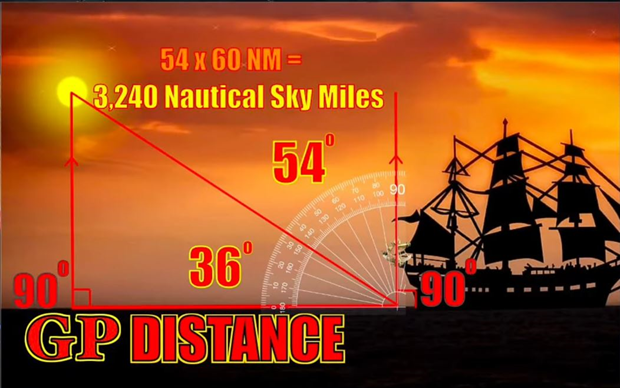And this is a modified version of the image, which illustrates the type of calculation I will use: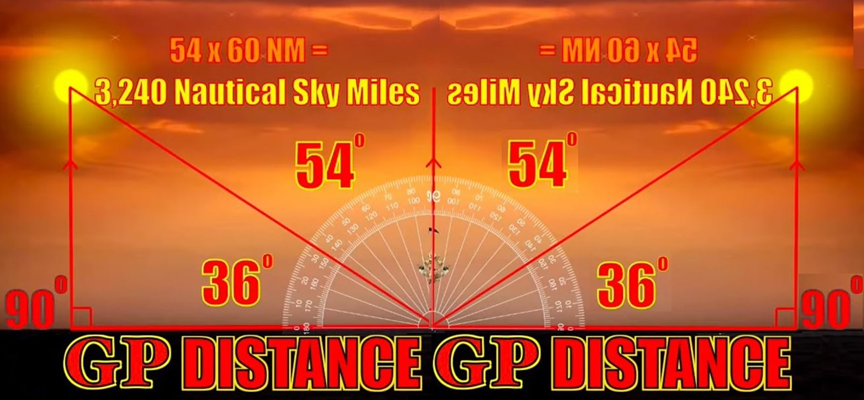I took 12 screenshots from my Nautical Almanac app. They involve 4 Geographical Positions (GP's):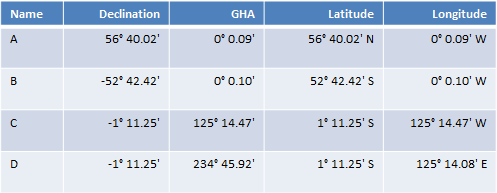My app won’t show the Latitude of Longitude of the substellar points, but it will show the Greenwich Hour Angle (GHA) and Declination, from which the GP can be derived. The relationship between them two should be obvious from the table. For point D, because the GHA is greater than 180°, the longitude East is calculated by 360° - 234° 45.92' = 125° 14.08'.

Each of the 4 GP’s is associated with a moment in time when a star is directly above that position:In celestial navigation, it is common to calculate the Height (=elevation angle) and Azimuth of a star from a certain Assumed Position and time. I have chosen positions halfway in between each combination of GP’s: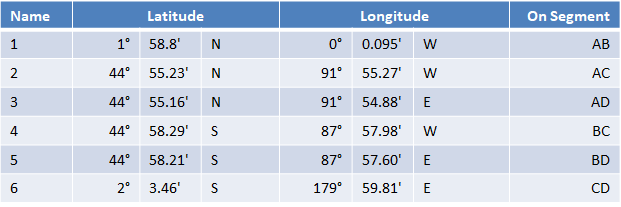Using Assumed Position 1 and the times for A (left) and B (right):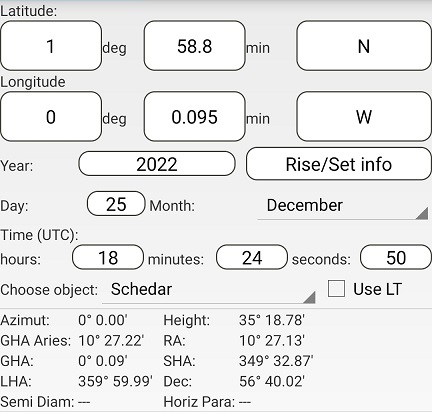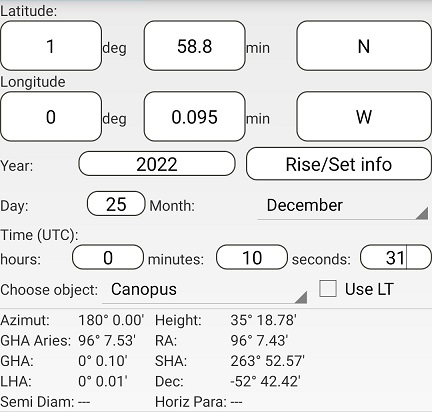The difference in azimuths is 180° - 0° = 180°.
The distance to both A and B is (90° - 35° 18.78') x 60 = 3281.22 nm
This gives AB = 3281.22 + 3281.22 = 6562.44 nm

Using Assumed Position 2 and the times for A (left) and C (right):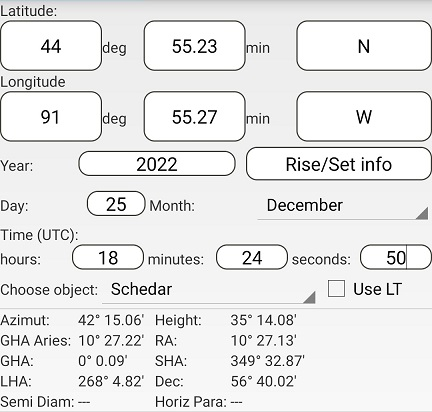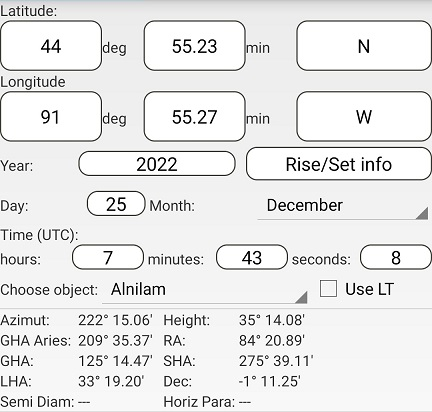The difference in azimuths is 222° 15.06' – 42° 15.06' = 180°
The distance to both A and C is (90° - 35° 14.08') x 60 = 3285.92 nm
This gives AC = 3285.92 + 3285.92 = 6571.84 nm

Using Assumed Position 3 and the times for A (left) and D (right):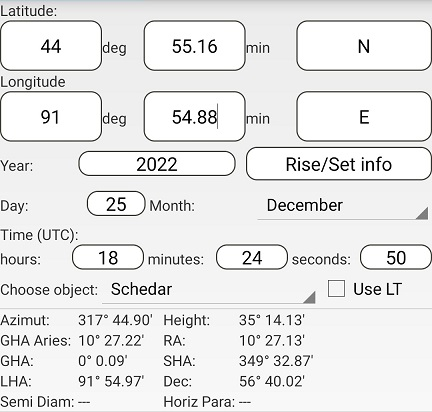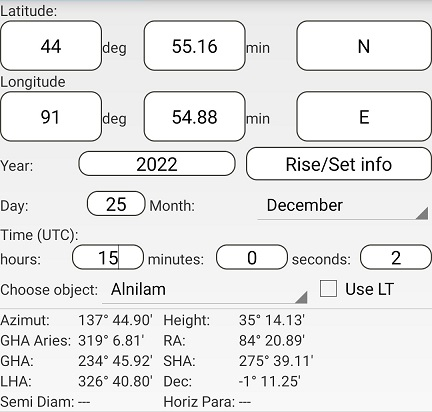The difference in azimuths is 317° 44.90' – 137° 44.90' = 180°
The distance to both A and D is (90° - 35° 14.13’) x 60 = 3285.87 nm
This gives AD = 3285.87 + 3285.87 = 6571.74 nm

Using Assumed Position 4 and the times for B (left) and C (right):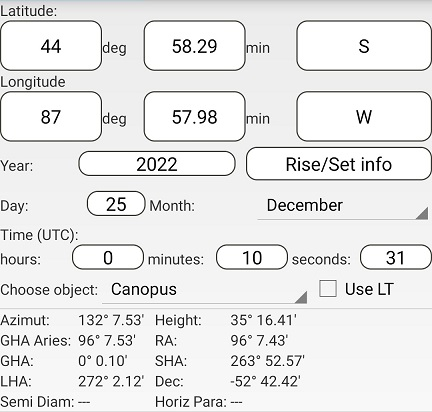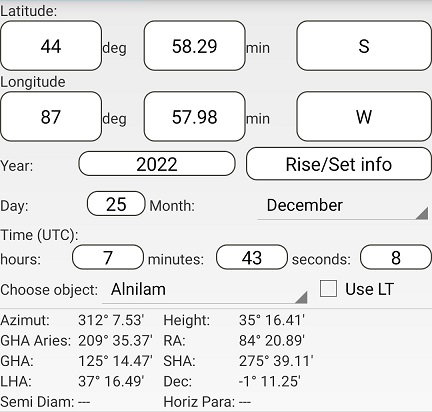The difference in azimuths is 312° 7.53' – 132° 7.53' = 180°
The distance to both B and C is (90° - 35° 16.41') x 60 = 3283.59 nm
This gives BC = 3283.59 + 3283.59 = 6567.18 nm

Using Assumed Position 5 and the times for B (left) and D (right):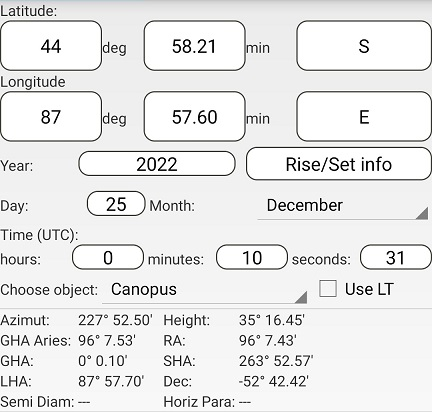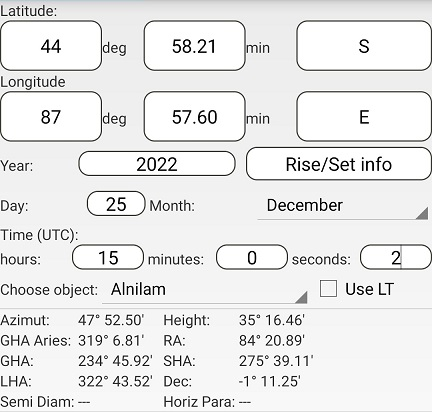The difference in azimuths is 227° 52.50' – 47° 52.50' = 180°
The distance to B is (90° - 35° 16.45') x 60 = 3283.55 nm
The distance to D is (90° - 35° 16.46') x 60 = 3283.54 nm
This gives BD = 3283.55 + 3283.54 = 6567.09 nm

Using Assumed Position 6 and the times for C and D: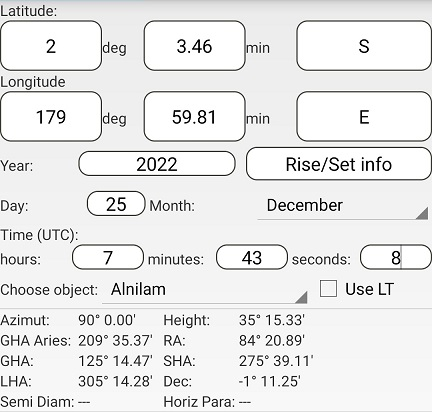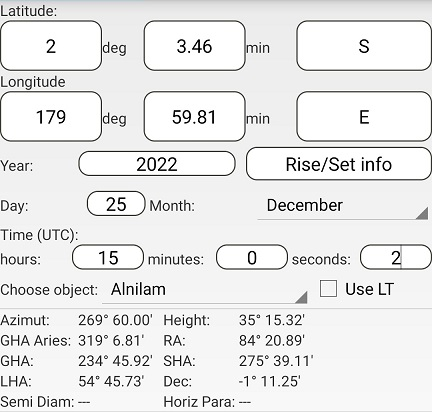The difference in azimuths is 270° - 90° = 180°
The distance to C is (90° - 35° 15.33') x 60 = 3284.67 nm
The distance to D is (90° - 35° 15.32') x 60 = 3284.68 nm
This gives CD = 3284.67 + 3284.68 = 6569.35 nm

In summary, we found the following distances:

AB = 6562
AC = 6572
BC = 6567
BD = 6567
CD = 6569

If we try to make a map of the flat earth that this is supposedly based on, with accurate distances, once we have placed A, B and C on it, we can’t find a place for point D such that the distances to the other 3 points are correct: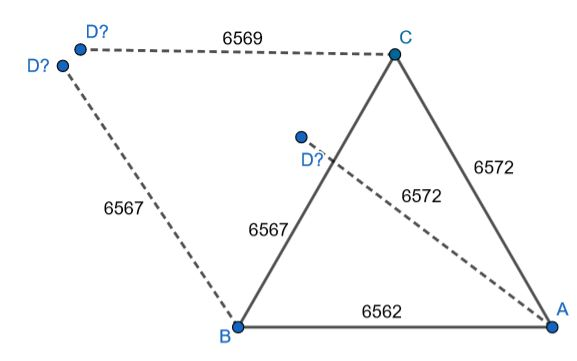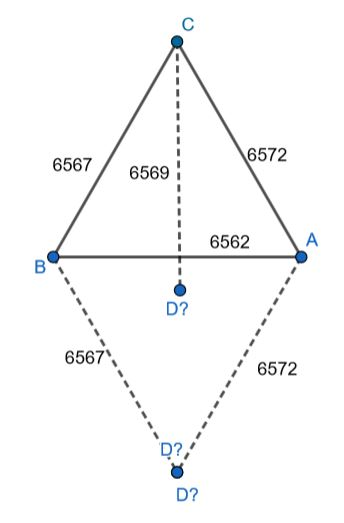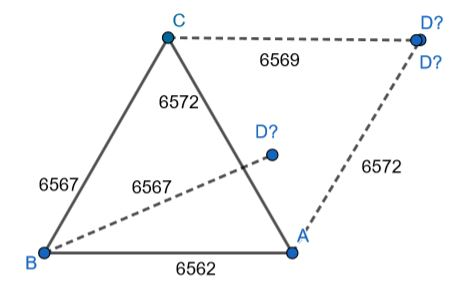The 6 distances between these 4 points, being impossible on a flat surface, contradict the claim that a Flat Earth is being used as the basis for celestial navigation.

#### Mendel

##### Senior Member.
Understanding the maths behind all of this is challenging.
Most people find this challenging, and Nathan Oakley is among them. That's why his argument isn't anything as complex as that. He has reiterated many times, in livestreams and shorts edited from them, that sextants measure elevation from a "flat baseline", which purportedly proves Earth is flat.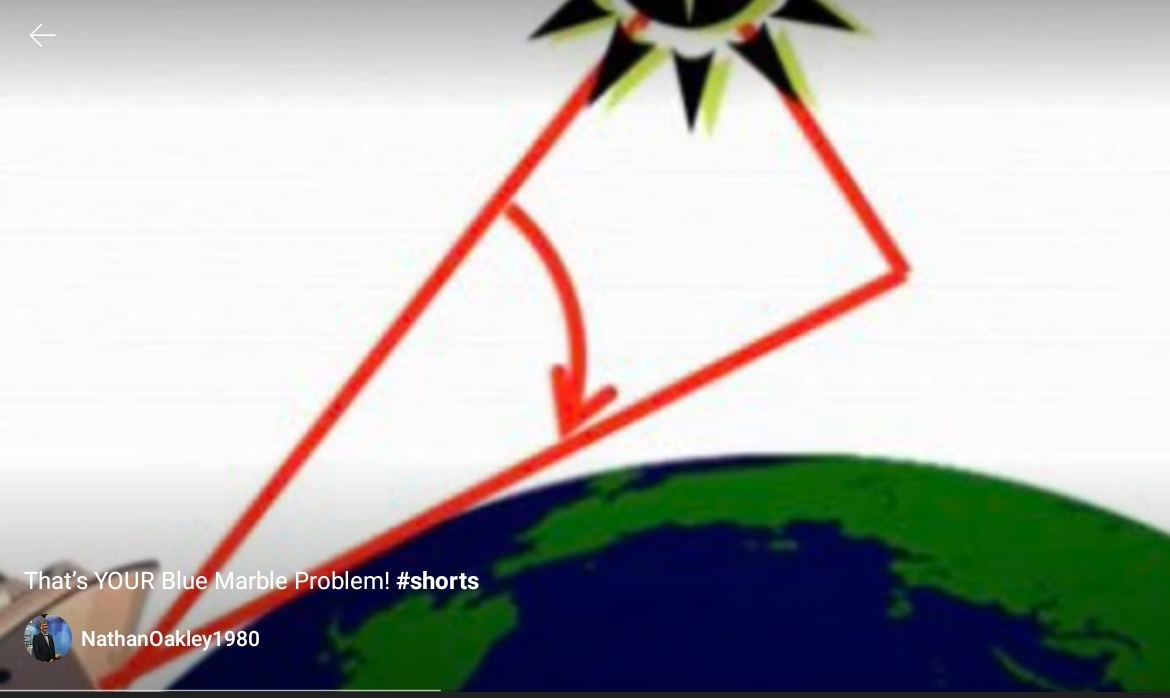There are two obvious counterarguments:
1) tangents on circles and spheres exist
2) you can do celestial navigation with zenith angles
Either of these suffices.

A zenith angle is the opposite of an elevation, where straight overhead=0⁰ zenith angle, and 40⁰ zenith angle=60⁰ elevation.

I will use 2 main premises to analyze the numbers from the app:
• The distance to a Geographical Position (GP) is the Zenith Distance multiplied by 60 nm. The zenith distance will be calculated as 90° – Height. Note that ‘Height’ means ‘Elevation angle’ in this context.
Article:
For navigation by celestial means when on the surface of the earth for any given instant in time a celestial body is located directly over a single point on the Earth's surface. The latitude and longitude of that point is known as the celestial body's geographic position (GP), the location of which can be determined from tables in the nautical or air almanac for that year.

What we need for a triangulation is the distances to 3 known points. Below, I'll explain how to get the distance to one GP; repeat 3 times and we're done (in principle).

Since the light from astronomical objects comes from so far away, it's essentially parallel everywhere on Earth, and that gives us the GP distance from the zenith angle.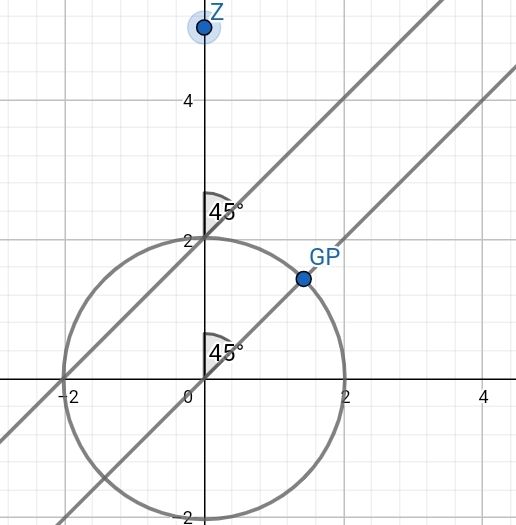In my geogebra diagram, the distance between the observer at the "top" of the circle and the GP is proportional to the size of the angle at the center of the Earth:
45⁰×60nm = 2700 nm (nautical miles) = 5000 km = 3107 statute miles.

The hard mathematics is figuring the ground points from the astronomical tables, and calculating where you are once you have the 3 points and distances. (If you had 3 GPs and distances on Flat Earth, you'd do similar maths to find your position.)

However, there is no way on Flat Earth to get a distance from an angle observation like I show above, because on Flat Earth, either the light comes in parallel (then it's at the same angle everywhere), or it doesn't and then you need the height of the astronomical object (star), but the astronomical tables don't give you that (it's impossible).

So, not only does Nathan Oakley's objection evaporate completely when you use zenith angles, looking more closely also reveals that celestial navigation (as practiced successfully for centuries of seafaring) can't work on a flat Earth.

#### Attachments

• zenith1.ggb
34.5 KB · Views: 27
Last edited:

#### MyMatesBrainwashed

##### Active Member
He has reiterated many times, in livestreams and shorts edited from them, that sextants measure elevation from a "flat baseline", which purportedly proves Earth is flat.
I've wondered if that would mean you can't measure angles when sat on a unicycle, or resting on any type of wheel.

Kind of easy to show that angles measured when not on a wheel are the same as measured when on a wheel. But I do like the idea of an angle measuring device going all whappy if it was on a wheel.

But to be honest I dunno if that's the crux of the argument.

#### JMartJr

##### Senior Member
This is a remarkably well-chosen flat earther argument, though. To explain what they claim they are proving, they use enough math for the average potential victim's eyes to glaze over but hopefully produce an impression of the conman knowing what he is talking about because look at all that math. To come along later and then show why they are wrong requires MORE math resulting in more glazed eyes, at which point the victim is left with "well, I guess I don't really understand it, but it's easier to continue believing what the first guy told me than to have to admit I was fooled."